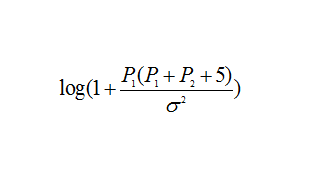# How to do it with CVX

I want to find the energy efficiency, how to do it with CVX？P 1 and P 2 are variables, and the rest are constants。Let’s consider the special case in which P2 = 0, and is not a variable.

Then the expression is convex for x^2 \le \sigma^2, and concave for x^2 \ge \sigma^2. So it is neither convex nor concave.over the entirety of its domain…

If I want to solve this problem. Can I transform it and use CVX？P 1 and P 2 are variables, and the rest are constants.

Due to the product terms (because P_R involves a sum of P_1 and P_2, you will have the same kind of non-convexity as in your first post.

You need to prove convexity, not just throw “random” problems out there, and find which ones forum readers come up with solutions for.